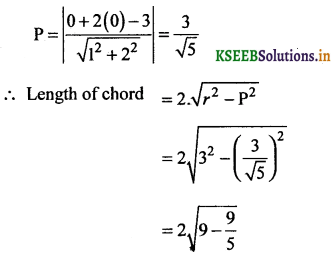# 2nd PUC Basic Maths Question Bank Chapter 15 Circles

Students can Download Basic Maths Question Bank Chapter 15 Circles Questions and Answers, Notes Pdf, 2nd PUC Basic Maths Question Bank with Answers helps you to revise the complete Karnataka State Board Syllabus and to clear all their doubts, score well in final exams.

## Karnataka 2nd PUC Basic Maths Question Bank Chapter 15 Circles

### 2nd PUC Basic Maths Circles One Mark Questions and Answers

Question 1.
Find the equation of the circle with centre at orgin and radius 4 units.
x2 + y2 = 42
x2 + y2 = 16

Question 2.
Find the equation of the circle with centre at (-3,2) and radius 5 units.
(x + 3)2 + (y – 2)2 = 52.

Question 3.
Write the equation of the circle with centreat (1,1) and radius 2 units
(x – 1)2 + (y – 1)2 = (√2)2
(x – 1)2 + (y – 1)2 = 2Question 4.
Find the equation of the point circle with centre at (a) (4, -5), (b) (-3,2), (c) (1,0)
(a) (x – 4)2 + (y + 5)2 = 0
(b) (x + 3)2 + (y – 2)2 = 0
(c) (x – 1)2 + y2 = 0

Question 5.
Find the centre of the circle x2 + y2 – 4x – 6y + 1 = 0.
Centre = (2, 3)

Question 6.
Find the radius of the circle x2 + y2 + 4x – 2y – 4 = 0.
g = 2, f = – 1, C = – 4.
r =$$\sqrt{g^{2}+f^{2}-C}$$ =$$\sqrt{4+1+4}$$ = √9 = 3.

Question 7.
Find the centre of the circle 4X2 + 4y2 – 15x – 18y + 11 = 0.
Divide the circle of by 4
x2 + y2 – $$\frac{15}{4}$$x – $$\frac { 18 }{ 4 }$$y + $$\frac { 11 }{ 4 }$$ = 0
Centre = $$\left(\frac{15}{8}, \frac{9}{4}\right)$$

Question 8.
Find the equation of the circle having the centre at (3,4) and touching the x-axis.
Given g = – 3, f=- 4 and c = g2 = 9 as ittouchs x-axis.
∴ Eqn. of the circle is x2 + y2 + 2gx + 2fy + c = 0
x2 + y2 – 6x – 8y + 9 = 0.Question 9.
Find the length of the chord of the circle x2 + y2 – 6x + 15y -16 = 0 intercepted by the x- axis.
Length of the chord intercepted by x-axis is 2$$\sqrt{g^{2}-c}$$
= 2$$\sqrt{9-(-16)}$$ = 2$$\sqrt{25}$$ = 2.5 = 10.

Question 10.
Find the equation of a circle whose centre is (4,2) and touchs the y-axis.
If the circle touchess the y-axis then f2 = c
The eqn. of the circle is x2 + y2 + 2gx + 2fy + c = 0
With g = – 4, f = – 2, c = f2 = 4 is x2 + y2 – 8x – 4y + 4 = 0.

Question 11.
Find the length of the chord of the circle x2 + y2 + 3x – 2 = 0 intercepted by y-axis.
Given f = 0, c = – 2
The length of the chord intercepted by y-axis is given by = 2$$\sqrt{f^{2}-c}=2 \sqrt{0-(-2)}=2 \sqrt{2}$$

Question 12.
Write the unit circle with centre at the origin in.
x2 + y2 = 1.### 2nd PUC Basic Maths Circles Two Marks Questions and Answers

Question 1.
Find the centre and radius of the circle x2 + y2 + 4x + 2y – 1 = 0.
Centre = (- g, -f) = (-2, -1), c = – 1
r = $$\sqrt{g^{2}+f^{2}-c}=\sqrt{4+1-(-1)}=\sqrt{5+1}=\sqrt{6}$$

Question 2
Find the equation of the circle with A(x1, y1) and B(x2, y2) as the ends of the diameter.A(x1, y1) and B(x2, y2) be 2 given points
P(x, y) be any point on the circle join
AP and BP and AP is perpendicular to BP
∴∠APB = 90° (Angle in a semicircle)
∴ Slope of AB x slope of PB = – 1
$$\frac{y-y_{1}}{x-x_{1}} \times \frac{y-y_{2}}{x-x_{2}}$$ = -1 ⇒ (x – x1) (x – x2) + (y – y1)(y – y2) = 0 is the required equation.

Question 3.
Find the equation of the circle whose ends of diameter are (3,1) and (-4,2).
(x – x1) (x – x2) + (y – y1) (y – y2) = 0
given (X1, y1) = (3, 1), (x2, y2) = (-4,2)
∴ (x – 3)(x + 4) + (y – 1)(y – 2) = 0
⇒ x2 + y2 + x – 3y -10 = 0 is the required equation.

Question 4.
Find the equation of the circle with centre (4,3) and which passes through (0,0).
Given centre = (4, 3)
and r = distance between (4, 3) and (0, 0)
r = $$\sqrt{(4-0)^{2}+(3-0)^{2}}$$ = $$\sqrt{16+9}$$ = 5
∴The required equation of the circle with centre (4, 3) and radius = 5 units is
(x – 4)2 + (y – 3)2 = 52
x2 + y2 – 8x – 6y = 0.Question 5.
Find the equation of the circle whose two diameters are x + 2y = 3 and x – y = 6 and radius is 6 units.
Solving the 2 diameters we get the centre.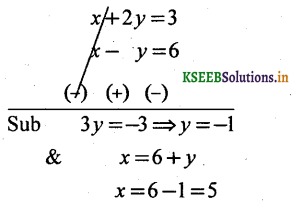∴The centre = (5, -1)andr = 6
Equation of circle is
(x – 5)2 + (y + 1)2 = 62
or x2 + y2 – 10x + 2y – 10 = 0.

Question 6.
If one end of the diameter of the circle x2 + y2 – 2x – 4y + 4 = 0 is (1,1). Find the other end.
Centre of the given circle = (1,2)
One end is given as A (1, 1)
Let the other end be B(x, y) = ?
W.K.T. centre = mid point of the diameter
∴(1,2) = $$\left(\frac{1+x}{2}, \frac{1+y}{2}\right)$$
⇒ 1 + x = 2 1 + y = 4
⇒ x = 1 y = 3
∴The other end of the diameter = B(l, 3).

Question 7.
Find the equation to the circle whose centre is same as the centre of the circle 2x2 + 2y2 – 6x + 8y – 3 = 0 and whose radius is 3 units.
Given circle 2x2 + 2y2 – 6x + 8y – 3 = 0
x2 + y2 – 3x + 4y – $$\frac { 3 }{ 2 }$$ = 0 .
The equation of the circle whose centre is same as the centre of x2 + y2 – 3x + 4y – $$\frac { 3 }{ 2 }$$ = 0 is of the form x2 + y2 – 3x + 4y + c = 0
g = $$\frac { -3 }{ 2 }$$, f = 2, c = $$\frac { -3 }{ 2 }$$
r = $$\sqrt{g^{2}+f^{2}-c}$$
S.B.S 3 = $$\sqrt{\frac{9}{4}+4-c}$$
9 = $$\frac{9+16-4 c}{4}$$
– 4c + 25 = 36
– 4c = 11 ⇒ C = $$\frac { 11 }{ -4 }$$
∴Required circle is x2 + y2 – 3x + 4y – $$\frac { 11 }{ 4 }$$
⇒ x2 + y2 – 3x + 4y – 11 =0.Question 8.
Find the equation of the circle passing through the points (1,0) (0,1) and (0,0).
Let the required circle is x2 + y2 + 2gx + 2fy + c = 0
This equation passing through (0, 0) (1, 0), (0, 1)
∴(0, 0) ⇒ c = 0 ‘
(1,0) ⇒ 1 – 2g = 0 ⇒ g = $$-\frac{1}{2}$$
(0, 1) ⇒ 1 + 2f = 0 ⇒ f = $$-\frac{1}{2}$$
∴ The equation of the circle is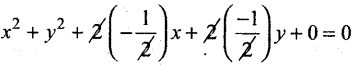x2 + y2 – x – y = 0.

Question 9.
Find the equation of the circle whose centre is same as the circle x2 + y2 – 2x + 4y -11 = 0 and the radius is 4 units.
Centre of the given circle is (1, -2)
∴The required circle with centre (1, -2) and r = 4 units is
(x – 1)2 + (y + 2)2 = 42
⇒ x2 + y2 – 2x + 4y – 11 = 0.

Question 10.
If (a, b) and (-5,1) are the ends of a diameter of the circle x2 + y2 + 4x – 4y – 2 = 0 find a ‘ and b.
Centre of the given circle = (- 2, 2)
W.k.t. centre = mid point of the diameter
(-2,2) = $$\left(\frac{a-5}{2}, \frac{b+1}{2}\right)$$
a – 5 = -4, b + 1=4
a = 1, b = 3
∴ (a, b) = (1, 3).

Question 11.
S.T. the line (3x – 4y + 6) = 0 touches the circle x2 + y2 – 6x + 10y – 15 = 0.
Centre of the given circle ~ (3,-5)
and r = $$\sqrt{g^{2}+f^{2}-c}=\sqrt{9+25-(-15)}=\sqrt{49}=7$$
Length of the perpendicular from the centre (3, -5) to the line 3x – 4y + 6 = 0 isLt. of perpendicular = radius = 7
∴The line touches the circle.Question 12.
If the radius of the circle x2 + y2 – 2x + 3y + k = 0 is $$\frac { 5 }{ 2 }$$ find k.
Centre of the given circle = $$\left(+1, \frac{-3}{2}\right)$$Question 13.
Find the value of k such that the line 3x – 4y + k = 0 may be a tangent to the circle x2 + y2 = 25
Centre of the circle = (0, 0)
Since the line is a tangent to the circle we get
radius = Length of the perpendicular from the centre (0, 0)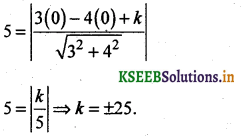Question 14.
Find A, if the line 3x + y + λ = 0 touches the circle x2 + y2 – 2x – 4y – 5 = 0.
Centre = (1, 2) and r = $$\sqrt{1+4+5}=\sqrt{10}$$
Since the line touches the circle ∴
radius = Length of the perpendicular.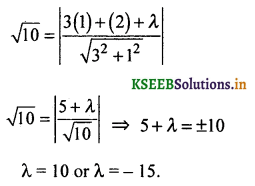Question 15.
Find the centre of the circle, two of the diameters are x + y = 2 and x – y = 0.
Point of intersection of the diameters is the centre
∴Solving the 2 diameters we get∴Center = (1,1)

Question 16.
Find the equation of the circle two of the diameters x + y = 4 and x – y = 2 and passing through the point (2, -1).
Point of intersection of two diameters is centre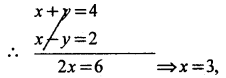y = 4 – x
= 4 – 3
= 1
∴The centre = (3,1).
radius = distance between the centre (3,1) and the point (2, -1)
r = $$\sqrt{(3-2)^{2}+(1+1)^{2}}$$
$$=\sqrt{1+4}=\sqrt{5}$$
∴Equation of the required circle with centre (3, 1) and r = √5 units is
(x – 3)2 + (y – 1)2 = (√5)
⇒ x2 + y2 – 6x – 2y + 5 = 0.

Question 17.
S.T. the circle x2 + y2 + 4x – 3y + 4 = 0 touches x-axis.
g = 2, c = 4
Condition for the circle to touch x-axis is g2 = c
Here 22 = 4 ⇒ 4 = 4
∴ The given circle touches x-axis.Question 18.
S.T. the circle x2 + y2 – 3x + 8y + 16 = 0 touches y-axis.
Here f = 4, c = 16
The condition for the circle to touch y-axis is f2 = c
Here 42 = 16 ⇒ 16 = 16
∴ The given circle touches y-axis.

Question 19.
S.T. the circle x2 + y2 – 2x + 2y + 1 = 0 touches both the co-ordinate axes.
The condition for the circle to touch both the axes.
is g2 = f2 = c
Here g = 1 f = – 1 and c = 1 .
∴ (1)2 = (-1)2 = 1 ⇒ 1 = 1 = 1
Hence the given circle touches both the axes.

Question 20.
Find the equation of the tangents to the circle x2 + y2 + 2x + 4y – 4 = 0 which are parallel to the line 5x + 12y + 6 = 0
Centre of the given circle = (-1, -2) and r = $$\sqrt{1+4+4}=\sqrt{9}$$ = 3
Equation of the tangent parallel to the given line can be taken as 5x + 12y + k = 0.
Length of parpendicur from (-1, -2) to the line 5x + 12y + k = 0∴ k – 29 = ±39 ⇒ k = 68 or -10
∴The equations of tangents are 5x + 12y + 68 = 0 and
5x + 12y – 10 = 0.Question 21.
Find the equation of the tangents to the circle x2 + y2 – 2x – 4y + 1 = 0 which are perpendicur to the line 3x – 4y – 7 = 0.
Centre (1,2), r = $$\sqrt{1+4-1}=\sqrt{4}$$ = 2
Any line ⊥lar to the given line can be .taken as 4x + 3y + k = 0
∴Length of the ⊥lar from (1,2) to the line 4x + 3y + k = 0 is⇒ 10 + k ± 10
⇒ k = 0 or – 20
∴The required equations are 4x + 3y = 0 and 4x + 3y – 20 = 0.

Question 22.
S.T. the line 1x + y = 4 passes through the centre of the circle x2 + y2 – 3x – 2y + 2 = 0.
Centre = $$\left(\frac{3}{2}, 1\right)$$
Put x = $$\frac{3}{2}$$, y = 1 in the equation 2x + y = 44 = 4
Thus the center $$\left(\frac{3}{2}, 1\right)$$ lies on the line 2x + y = 4.

### 2nd PUC Basic Maths Circles Five or Six Marks Questions and Answers

Question 1.
Find the equation of the circle passing through the points
(i) (2,0) (-1, 3) and (-2, 0).
Let the equation of the required circle is
x2 + y2 + 2gx + 2fy + c = 0 ‘
Given that this equation passes through the points.
(2, 0) ⇒ 4 + 0 + 4g + 0 + c = 0
4g + 4 + c = 0 _____ (1)
(-1,3) ⇒ 1 + 9 – 2g + 6f + c = 0
-2g + 6f + c + 10 = 0 ______ (2)
(-2,0) ⇒ 4 + 0 – 4g + c = 0
-4g + 4 + c = 0 (3)
Solving equations 1, 2 and 3 we get
put Eqn(3) in Eqn(l) i.e., 4 + c = 4g
we get c = – 4, g = 0, and f= – 1
∴ The required equation of the circle is x2 + y2 – 2y – 4 = 0.Question 2.
(1,1) (5,-5) and (6,-4).
Let the equation of the required circle is
x2 + y2 + 2gx + 2fy + c = 0
Given that this equation passes through the points.
(1,1) ⇒ 1 + 1 + 2g + 2f + c
2g + 2f + c + 2 = 0 — (1)
(5,-5) ⇒ 25 + 25 + 10g – 10f + c = 0
10g – 10f + c + 50 = 0 (2)
(6, -4) ⇒ 36 + 16 + 12g – 8f + c = 0
I2g – 8f + c + 52 = 0 (3)
Solving equations 1, 2 and 3 we get
g = -3, c = 0, f = 2
∴The required equation of the circle is x2 + y2 – 6y + 4y = 0.

Question 3.
Find the equation of a circle passing through (0,0) (0,2) and (- 3, -1).
Let the required equation of the circle be
x2 + y2 + 2gx + 2fy + c = 0
This equation passes through the points.
(0, 0) ⇒ 0 + 0 + 0 + c – 0 ⇒ c = 0 ______ (1)
(0, 2) ⇒ 0 + 4 + 0 + 4f + c = 0 ⇒ 4f + 4 = 0 ______ (2)
f = -1
(-3, -1) ⇒ 9 + 1 – 6g – 2f + c = 0 ⇒ -6g + 2 + 10 = 0
– 6g = – 12
g = 2 ______ (3)
∴Required equation of the circle is x2 + y2 + 4x – 2y = 0.

Question 4.
A circle has its centre on the y-axis and passess through (- 1, 3) and (2, 5). Find its equation.
Let the required equation of the circle be
x2 + y2 + 2gx + 2fy + c = 0
Given the the centre (- g, – f) lies on the y-axis.
∴x co-ordinate of the centre is 0 ⇒ g = 0 _______ (1)
Also given the required equation passess through the points (-1,3) and (2, 5)
(-1,3) ⇒ (-1)2 + (3)2 + 2(-1)g + 2(3)f + c = 0
-2g + 6f + c + 10 = 0 (g = 0)
∴6f + c + 10 = 0 _______ (2)
(2,5) ⇒ 22 + 52 + 2g(2) + 2f(5) + c = 0
4g + 10f + 29 + c = 0
∴10f + 29 + c = 0 _______ (3)
Solving equations 2 and 3 we get f = $$-\frac{19}{4}$$ & c = $$\frac { 37 }{ 2 }$$
we get g = 0, f = $$\frac{19}{4}$$, c = $$-\frac{37}{2}$$
∴Required equation of the circle is2x2 + 2y2 – 19y + 37 = 0.Question 5.
Find the equation of a circle passing through (0,0) (1,1) and has its centre on x-axis.
Let the required equation of the circle is
x2 + y2 + 2gx + 2fy + c = 0
Given the the centre (- g, -f) lies on the x-axis ⇒ f = 0 _______ (1)
Also this equation passess through the points (0, 0) and (1,1)
(0,0) ⇒ c = 0 _______ (2)
(1, 1) ⇒ 1 + 1 + 2g = 0 ⇒ g = -1 _______ (3)
The required equation of the circle is x2 + y2 – 2x = 0.

Question 6.
Find the equation of the circle passing through the points (0,4) and (4,7) and its centre lies on the line 3x + 4y = 7.
Le2 + y2 + 2gx + 2fy + c = 0
Given this circle passess through the points (0, 4) and (4, 7)
(0,4) ⇒ 16 + 8f + c = 0 _______ (1)
(4,7) ⇒ 8g + 14f + c = – 65 _______ (2)
Also the centre (- g, – f) lies on the line 3x + 4y = 7
∴ – 3g – 4f = 7 _______ (3)
Solving equations 1, 2, and 3 we get
g = – 11, f = $$\frac { 13 }{ 2 }$$, c = -68
The required equations of the c ircle is
∴x2 + y2 – 22x + 13y – 68 = 0.Question 7.
S.T. the line 3x – 4y – 20 = 0 touches the circle x2 + y2 – 2x- 4y — 20 = 0 and also find the point contact.
The centre of the given circle = (1,2) and r = $$\sqrt{1^{2}+2^{2}-(-20)}=\sqrt{1+4+20}=\sqrt{25}$$ = 5
Condition is
Length of the ⊥lar from the centre (1, 2) to the line = radius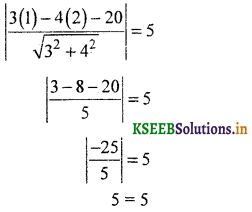5 = 5
∴ The line 3x – 4y – 20 = 0 touches the circle
Any line perpendicular to 3x – 4y – 20 = 0 is of the from
4x + 3y + k = 0 but it passess through (1,2)
4 + 6 + k = 0 ⇒ k = — 10
∴ Equation of the ⊥lar line is 4x + 3y- 10 = 0
The point of interesection of the lines 4x + 3y – 10 = 0 and 3x – 4y -20 = 0.
Solving the above equations we get
x = 4 and y = – 2
∴ The point of contact is (4, -2).

Question 8.
Find the equation of the circle which passess through (2, 3). having its centre on the je-axis and radius 5 units.
Let the required equation of the circle is x2 + y2 + 2gx + 2fy + c = 0
This passess through the point (2, 3) we get
4 + 9 + 4g + 6f + c = 0
4g + 6f + c + 13 = 0 _____ (1)
Centre (- g, -f) lies on the x axis ⇒ f = 0 _____ (2)
Also given r = 5 units
$$\sqrt{g^{2}+f^{2}-c}=5$$ = 5 ⇒ g2 + f2 – c = 25
g2 – c = 25 _____ (3)
from eqn.(l) we get .
4g + 13 = – c
Eqn(3) gives.
g2 + 4g + 13 = 25
g2 + 4g – 12 = 0
(g + 6)(g – 2) = 0
g = – 6 or 2
when g = – 6, c = 11
when g = 2 c = – 21
∴ The required equations of the circles are
x2 + y2 – 12x + 11 = 0
x2 + y2 + 4x – 21 = 0.Question 9.
S.T. the points (1,1) (- 2,2) (- 6,0) and (- 2, – 8) are concyclic.
First find the equation of the circle passess through the points (1, 1) (-2, 2) and (- 6, 0). If the 4th point (-2, -8) satisfies the circle then the points are concyclic
Let equation of the required circle is
x2 + y2 + 2gx + 2fy + c = 0
This equation passess through the point (1, 1) (-2, 2) and (-6, 0)
(1, 1) ⇒ 2g + 2f + c + 2 = 0 _____ (1)
(-2, 2) ⇒ 4g + 4f + c = -8 _____ (2)
(-6,0) ⇒ -12g + c = – 36 _____ (3)
Eqn. 1 – 2 gives 3g – f = 3 _____ (4)
Eqn. 2 – 3 gives 2g + f = 7 _____ (5)
Adding eqns. 4 and 5 we get
5g = 10 ⇒ g = 2
f = 7 – 2g = 7 – 4 = 3
f = 3
2g + 2f + c = -2
c = – 2 – 2g – 2f
= – 2 – 4 – 6
c = – 12
The equation of the circle is x2 + y2 + 4x + 6y – 12 = 0
put x = – 2, and y = – 8 in the above circle ‘
we get (-2)2 + (-8)2 + 4(-2) + 6(—8) -12 = 0
4 + 64 – 8 – 48 – 12 = 0
68 – 68 = 0
0 = 0
∴ The 4 points are concyclic.

Question 10.
S.T. the following points arc concyclic (0,0) (1,1) (5, -5) and (6, -4).
Let the equation of the circle passing through
(0, 0) (1, 1) and (5,-5) be x2 + y2 + 2gx + 2fy + c = 0
It passes through (0,0) ⇒ c = 0 _____ (1)
It passes through (1, 1) ⇒ 2g + 2f + c + 2 = 0
2g + 2f + 2 = 0
g + f + 1 = 0 _____ (2)
It passes through (5,-5) ⇒ 10g – 10f + 50 = 0
g – f + 5 = 0 _____ (3)
Solving 2 and 3 we get g = – 3 and f = 2
Thus, the equation of the circle = x2 + y2 – 6x + 4y = 0
Put x = 6 and y = – 4 in L.H.S of the above eqn.
36 + 16 – 36 – 16 = 0
0 = 0
Thus, the point (6, -4) lies on the circle.
Hence, the given 4 points lie on the circle and concyclic.Question 11.
Find the length of the chord intercepted by the circle x2 + y2 – 8x – 6y = 0 and the line x – 7y – 8 = 0
Centre of the given circle = (4, 3)
and r = $$\sqrt{16+9-0}$$ = 5
P = Length of ⊥lar from C (4, 3) to the line x – 7y – 8 = 0
Length of the chord∴ The length of the chord = 5√2Question 12.
Find the length of the chord intersepted by the the circle x2 + y2 = 9 and the line x + 2y = 3.